Next: Analytic solution Up: The chaotic pendulum Previous: The chaotic pendulum

## Introduction

Up to now, we have mostly dealt with problems which are capable of analytic solution (so that we can easily validate our numerical solutions). Let us now investigate a problem which is quite intractable analytically, and in which meaningful progress can only be made via numerical means.

Consider a simple pendulum consisting of a point mass, at the end of a light rigid rod of length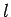, attached to a fixed frictionless pivot which allows the rod (and the mass) to move freely under gravity in the vertical plane. Such a pendulum is sketched in Fig. 21. Let us parameterize the instantaneous position of the pendulum via the anglethe rod makes with the downward vertical. It is assumed that the pendulum is free to swing through 360 degrees. Hence,and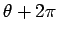both correspond to the same pendulum position.

The angular equation of motion of the pendulum is simply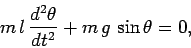(72)

where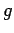is the downward acceleration due to gravity. Suppose that the pendulum is embedded in a viscous medium (e.g., air). Let us assume that the viscous drag torque acting on the pendulum is governed by Stokes' law (see Sect. 3.12) and is, thus, directly proportional to the pendulum's instantaneous velocity. It follows that, in the presence of viscous drag, the above equation generalizes to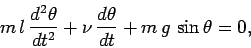(73)

whereis a positive constant parameterizing the viscosity of the medium in question. Of course, viscous damping will eventually drain all energy from the pendulum, leaving it in a stationary state. In order to maintain the motion against viscosity, it is necessary to add some external driving. For the sake of simplicity, we choose a fixed amplitude, periodic drive (which could arise, for instance, via periodic oscillations of the pendulum's pivot point). Thus, the final equation of motion of the pendulum is written(74)

whereandare constants parameterizing the amplitude and angular frequency of the external driving torque, respectively.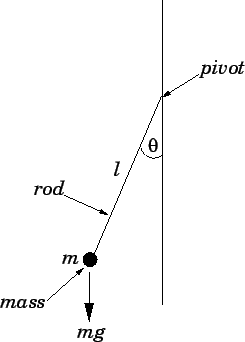Let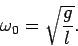(75)

Of course, we recognizeas the natural (angular) frequency of small amplitude oscillations of the pendulum. We can conveniently normalize the pendulum's equation of motion by writing,(76)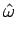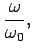(77)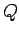(78)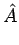(79)

in which case Eq. (74) becomes(80)

From now on, the hats on normalized quantities will be omitted, for ease of notation. Note that, in normalized units, the natural frequency of small amplitude oscillations is unity. Moreover,is the familiar quality-factor--roughly, the number of oscillations of the undriven system which must elapse before its energy is significantly reduced via the action of viscosity. The quantityis the amplitude of the external torque measured in units of the maximum possible gravitational torque. Finally,is the angular frequency of the external torque measured in units of the pendulum's natural frequency.

Equation (80) is clearly a second-order o.d.e. It can, therefore, also be written as two coupled first-order o.d.e.s: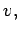(81)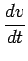(82)Next: Analytic solution Up: The chaotic pendulum Previous: The chaotic pendulum
Richard Fitzpatrick 2006-03-29